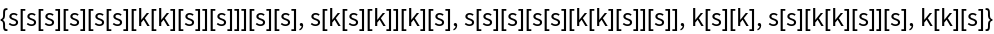#Function Repository Resource:

# CombinatorMatches

Get an association of match positions to rule matches, sorted according to traversal scheme, for a combinator expression

Contributed by: Wolfram Research
 ResourceFunction["CombinatorMatches"][cmb,scheme] gives an association of match positions to rule matches for S and K combinator transformations, sorted by scheme, for combinator expression cmb. ResourceFunction["CombinatorMatches"][rules,cmb,scheme] gives an association of match positions to rule matches for rules, sorted by scheme, for combinator expression cmb.

## Details and Options

ResourceFunction["CombinatorMatches"] finds rule matches, instances where the rules can be applied to parts of cmb. The keys of the association are the positions of the rule matches within cmb. The values of the association refer to the index in rules of the applicable combinator transformations.
The argument cmb must be given as a combinator expression in nested bracket form.
In scheme, both direction ("Leftmost" or "Rightmost") and depth order ("Outermost" or "Innermost") must be specified, in order of their priority as sorting criteria.
The scheme, which is a list, can include an optional third element, specified as an integer number or Infinity, that indicates how many elements from the sorted list should be given.
The combinator transformations of interest should be given as a list of Rule or RuleDelayed elements in rules.
ResourceFunction["CombinatorMatches"] takes the following option:
 SKGlyphs symbols used to specify default transformation rules

## Examples

### Basic Examples (5)

Find the leftmost-outermost-sorted matches of the combinator expression in the SK basis:

 In:=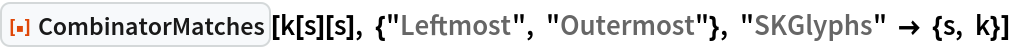Out=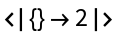Find the leftmost-outermost-sorted matches of the combinator expression in the SK basis:

 In:=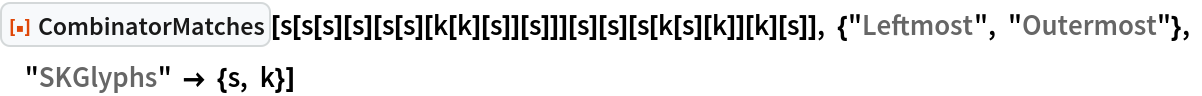Out=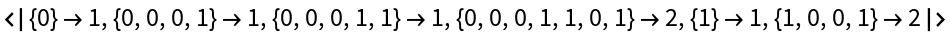Sort the SK basis matches of the expression in outermost-leftmost order:

 In:=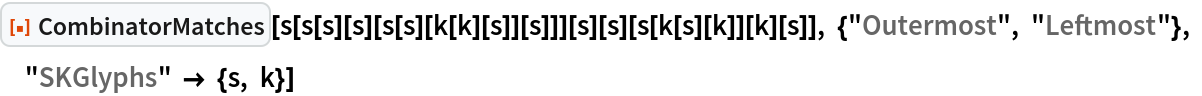Out=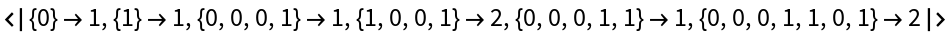Choose the first two SK basis matches of the expression from the innermost-rightmost evaluation order:

 In:=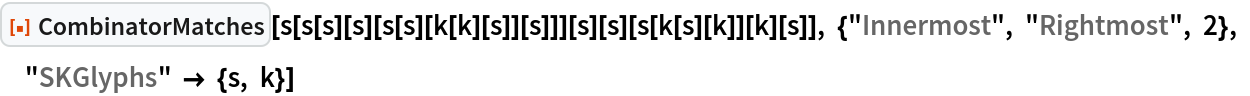Out=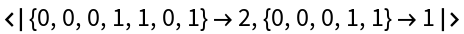Find K combinator matches of an expression in rightmost-outermost order:

 In:=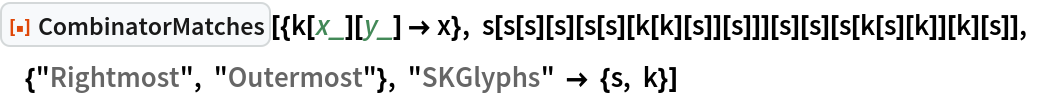Out=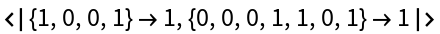### Scope (2)

Group the match positions by the corresponding rule:

 In:=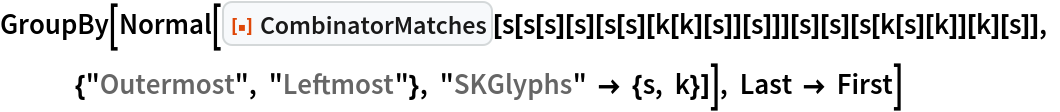Out=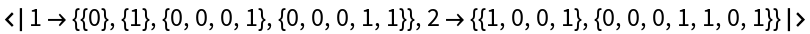Extract rule-matched subexpressions of a combinator expression:

 In:=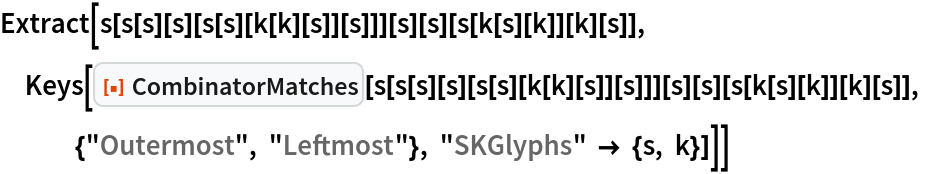Out=